In the binomial distribution, there are a number of probabilities, like:

• the trial number is indeterminately large, e., n -> ∞
• porq is minor, then the controlling form of the binomial distribution is known as ‘normal distribution’. It is thus a continuous distribution and the probability density function is given by,

f(x) = 1/ σ √2π. e-(x-µ)2/2σ2,                       -∞<x<∞, -∞ <µ<∞

= 1/ σ √2π. exp [ – (x-µ)2/2σ2]

It is thus represented by N (11, cr2) where 1.1. = Mean and cr2 = Variance.

Thisarrangement was established by Carl Friedrich Gauss, which is also known as ‘Gaussian Distribution’.

Properties

(i) The normal curve is bell shaped and symmetrical about the .line x = µ.

(ii) Median: Let M be the median, then

Mʃ-∞f(x) dx = 1/ 2

= 1/ σ √2π Mʃ-∞exp. [-(x-µ)2/2σ2]dx = 1/ 2

= 1/ σ √2π Mʃ-∞exp. [-(x-µ)2/2σ2]dx + 1/ σ √2π Mʃ-∞exp. [-(x-µ)2/2σ2]dx = ½

In the first integral we consider z= x-µ/σ, then what we obtain is

1/√2π ʃ0 exp. [-z2/2] dz = ½

So therefore, 1/ 2 + 1/ σ √2π Mʃµ exp. [-(x-µ)2/2σ2]dx = 1/ 2

1/ σ √2π Mʃµ exp. [-(x-µ)2/2σ2]dx =0

µ = M

(iii) Mode: It is the value of x for which f(x) is maximum i.e., f’ (x) = 0 and f” (x) < 0.

Therefore, we obtain, mode = µ

Note. Mean, Median and Mode might match.

(iv) Mean-deviation (M.D.)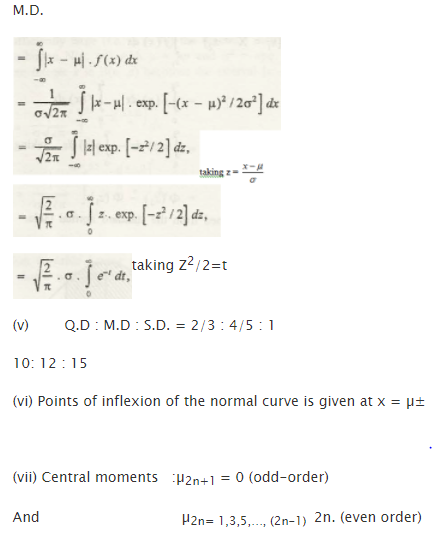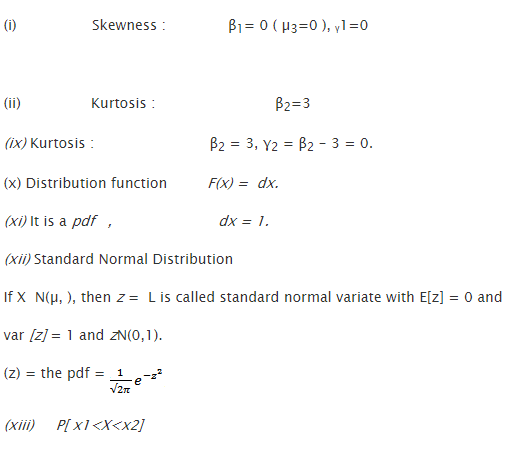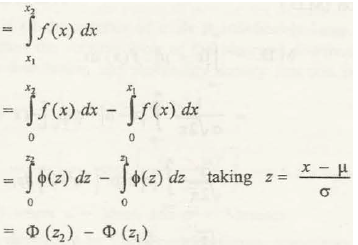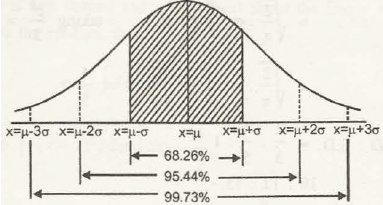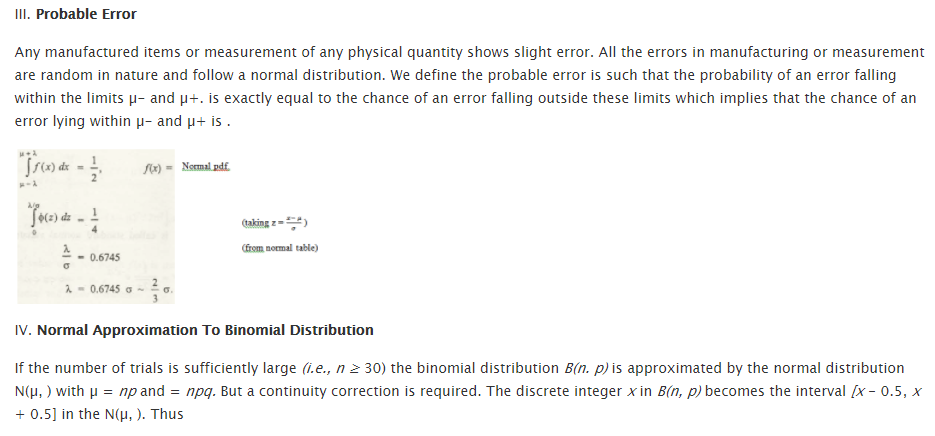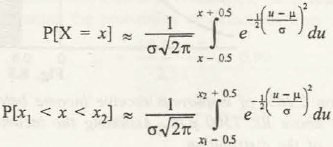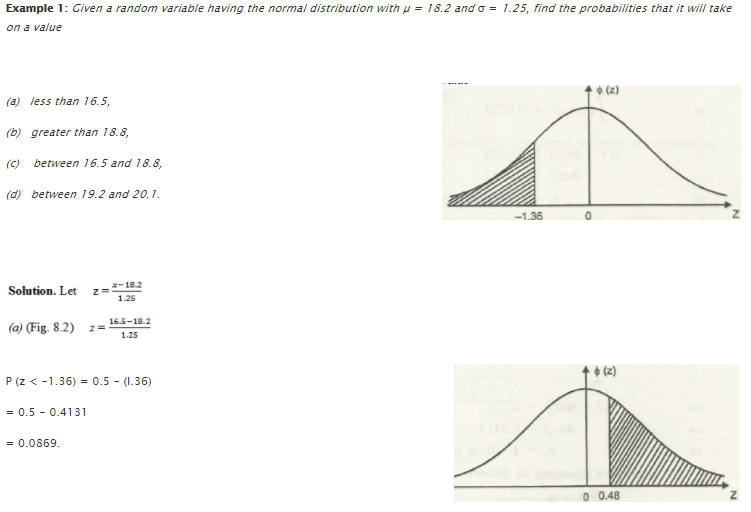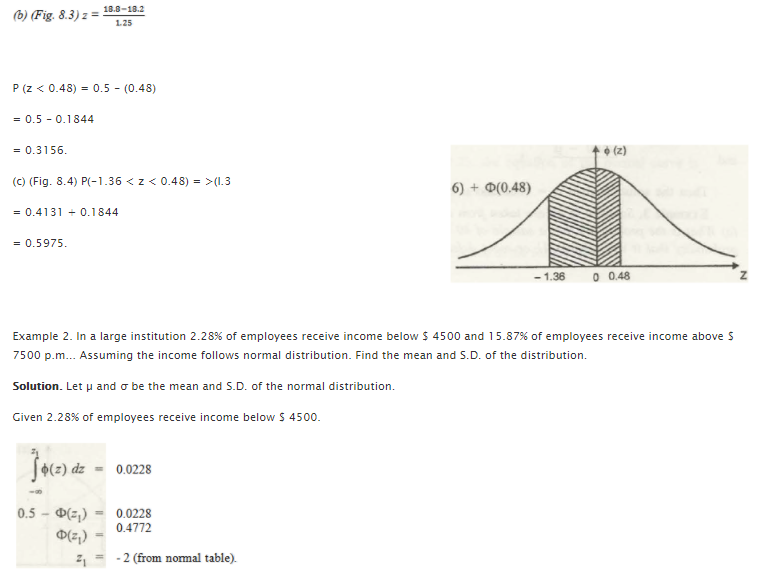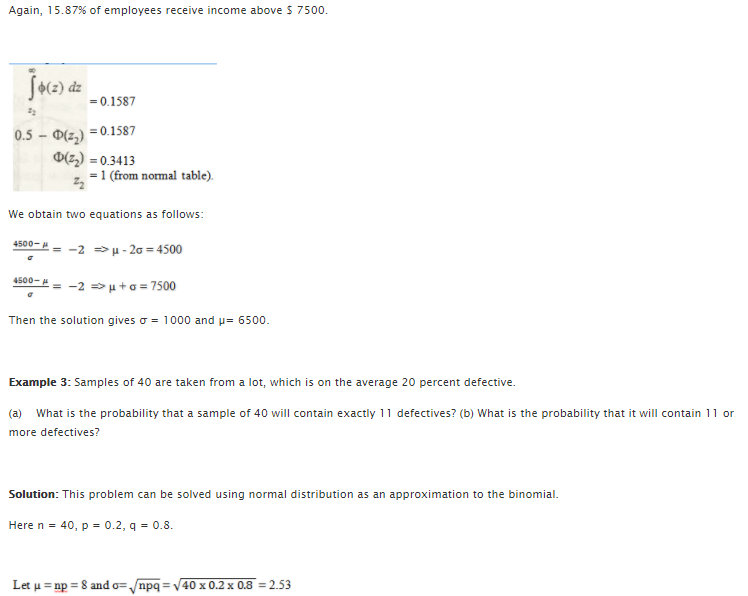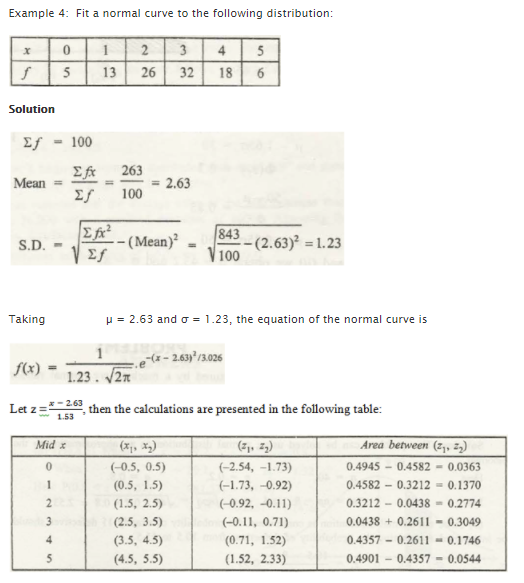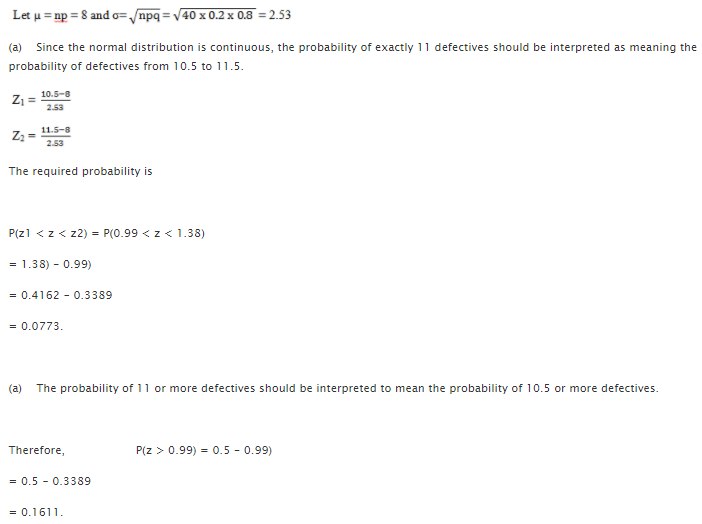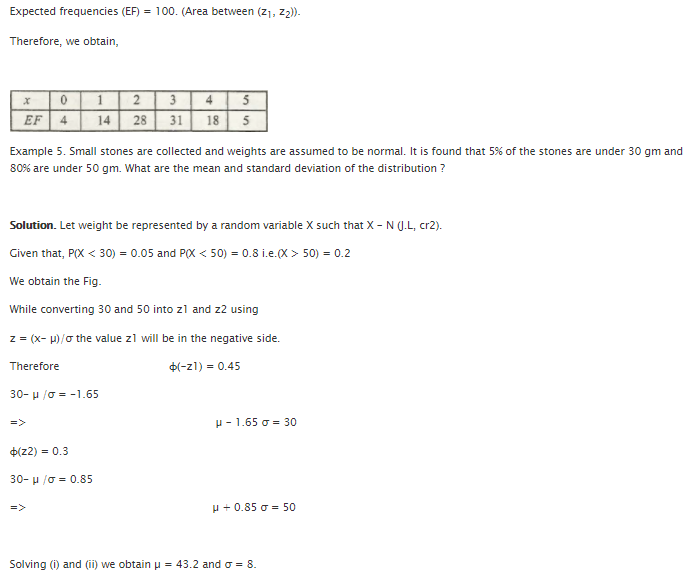### Customer Reviews

My Homework Help
Rated 5.0 out of 5 based on 510 customer reviews at